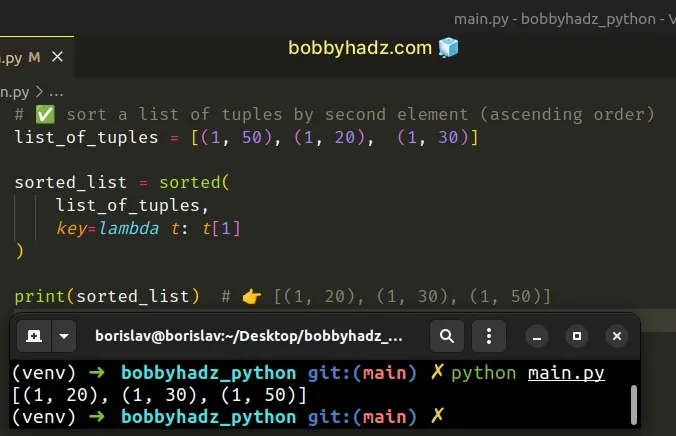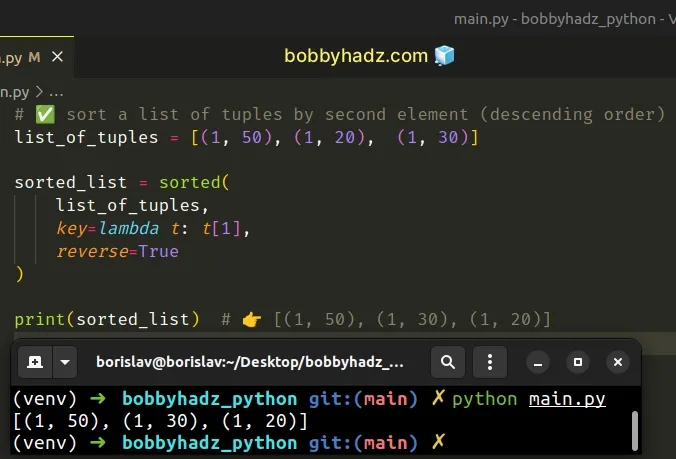# Sort List of Tuples by second or multiple elements in PythonLast updated: Feb 19, 2023
5 min## #Sort a list of tuples by the second element in Python

Use the `key` argument of the `sorted()` function to sort a list of tuples by the second element.

The function will return a new list, sorted by the second tuple element.

main.py
```Copied!```# ✅ sort a list of tuples by second element (ascending order)
list_of_tuples = [(1, 50), (1, 20),  (1, 30)]

sorted_list = sorted(
list_of_tuples,
key=lambda t: t
)

print(sorted_list)  # 👉️ [(1, 20), (1, 30), (1, 50)]
``````If you need to sort a list of tuples by the second element in descending order, use the following code sample instead.

main.py
```Copied!```# ✅ sort a list of tuples by second element (descending order)
list_of_tuples = [(1, 50), (1, 20),  (1, 30)]

sorted_list = sorted(
list_of_tuples,
key=lambda t: t,
reverse=True
)

print(sorted_list)  # 👉️ [(1, 50), (1, 30), (1, 20)]
``````The sorted function takes an iterable and returns a new sorted list from the items in the iterable.

The function takes an optional `key` argument that can be used to sort by different criteria.

main.py
```Copied!```list_of_tuples = [(1, 50), (1, 20),  (1, 30)]

sorted_list = sorted(
list_of_tuples,
key=lambda t: t
)

print(sorted_list)  # 👉️ [(1, 20), (1, 30), (1, 50)]
``````
Python indexes are zero-based. The first item in a tuple has an index of `0`, the second an index of `1`, etc.

The `key` argument can be set to a function that determines the sorting criteria.

The example sorts the list of tuples by the second element in each tuple.

## #Sort a list of tuples by the second element in descending order

If you need to sort the list of tuples by the second element in descending order (greatest to lowest), set the `reverse` argument to `true` when calling `sorted()`.

main.py
```Copied!```list_of_tuples = [(1, 50), (1, 20),  (1, 30)]

sorted_list = sorted(
list_of_tuples,
key=lambda t: t,
reverse=True
)

print(sorted_list)  # 👉️ [(1, 50), (1, 30), (1, 20)]
``````
If the `reverse` argument is set to `True`, then the elements are sorted as if each comparison were reversed.

## #Sort a list of tuples by the second element using itemgetter()

You can also use the `operator.itemgetter()` method to specify the index you want to sort by.

main.py
```Copied!```from operator import itemgetter

list_of_tuples = [(1, 50), (1, 20),  (1, 30)]

sorted_list = sorted(
list_of_tuples,
key=itemgetter(1)
)

print(sorted_list)  # 👉️ [(1, 20), (1, 30), (1, 50)]
``````

The operator.itemgetter method returns a callable object that fetches the item at the specified index.

For example, `x = itemgetter(1)` and then calling `x(my_tuple)`, returns `my_tuple`.

## #Sort a list of tuples by multiple elements in Python

To sort a list of tuples by multiple elements:

1. Pass the list to the `sorted()` function.
2. Use the `key` argument to select the elements at the specific indices in each tuple.
3. The `sorted()` function will sort the list of tuples by the specified elements.
main.py
```Copied!```list_of_tuples = [(1, 3,  100), (2, 3,  50), (3, 2, 75)]

# ✅ sort a list of tuples by second and third elements
sorted_list = sorted(
list_of_tuples,
key=lambda t: (t, t)
)

print(sorted_list)  # 👉️ [(3, 2, 75), (2, 3, 50), (1, 3, 100)]
``````

The sorted function takes an iterable and returns a new sorted list from the items in the iterable.

The function takes an optional `key` argument that can be used to sort by different criteria.

The `key` argument can be set to a function that determines the sorting criteria.

The example sorts the list of tuples by the elements at index `1` and `2` in each tuple.

Since the second item in the third tuple is the lowest, it gets moved to the front.

The second items in the first and second tuples are equal (both `3`), so the third items get compared and since `50` is less than `100`, it takes second place.

## #Sort a list of tuples by multiple elements in descending order

If you need to sort a list of tuples by multiple elements in descending order, set the `reverse` argument to `True` in the call to the `sorted()` function.

main.py
```Copied!```list_of_tuples = [(1, 3,  100), (3, 2, 75),  (2, 3,  50)]

sorted_list = sorted(
list_of_tuples,
key=lambda t: (t, t),
reverse=True
)

print(sorted_list)  # 👉️ [(1, 3, 100), (2, 3, 50), (3, 2, 75)]
``````
If the `reverse` argument is set to `True`, then the elements are sorted as if each comparison were reversed.

2 of the tuples have `3` as the second element, and since we set the `reverse` argument to `True`, the tuple with the greater 3rd element gets moved to the front.

The last tuple in the list is the one with the lowest second element.

## #Sort a list of tuples by multiple elements using operator.itemgetter

You can also use the `operator.itemgetter()` method to specify the indexes you want to sort by.

main.py
```Copied!```from operator import itemgetter

list_of_tuples = [(1, 3,  100), (2, 3,  50), (3, 2, 75)]

# ✅ sort list of tuples by second and third elements
sorted_list = sorted(
list_of_tuples,
key=itemgetter(1, 2),
)

print(sorted_list)  # 👉️ [(3, 2, 75), (2, 3, 50), (1, 3, 100)]
``````

The operator.itemgetter method returns a callable object that fetches the item at the specified index.

For example, `x = itemgetter(1)` and then calling `x(my_tuple)`, returns `my_tuple`.

Using the `itemgetter` method is faster than using a lambda function, but it is also a bit more implicit.

## #Sort a list of tuples by multiple elements using list.sort()

Alternatively, you can use the `list.sort()` method to sort the list of tuples by multiple elements in place.

main.py
```Copied!```from operator import itemgetter

list_of_tuples = [(1, 3,  100), (2, 3,  50), (3, 2, 75)]

list_of_tuples.sort(key=itemgetter(1, 2))

print(list_of_tuples)  # 👉️ [(3, 2, 75), (2, 3, 50), (1, 3, 100)]
``````

The list.sort method sorts the list in place and it uses only `<` comparisons between items.

The method takes the following 2 keyword-only arguments:

NameDescription
keya function that takes 1 argument and is used to extract a comparison key from each list element
reversea boolean value indicating whether each comparison should be reversed

Note that the `list.sort` method mutates the list in place and returns None.

You can use the `sorted()` function if you need a new list instance rather than an in-place mutation.

I've also written an article on how to get the indices of a sorted list.

I wrote a book in which I share everything I know about how to become a better, more efficient programmer.You can use the search field on my Home Page to filter through all of my articles.